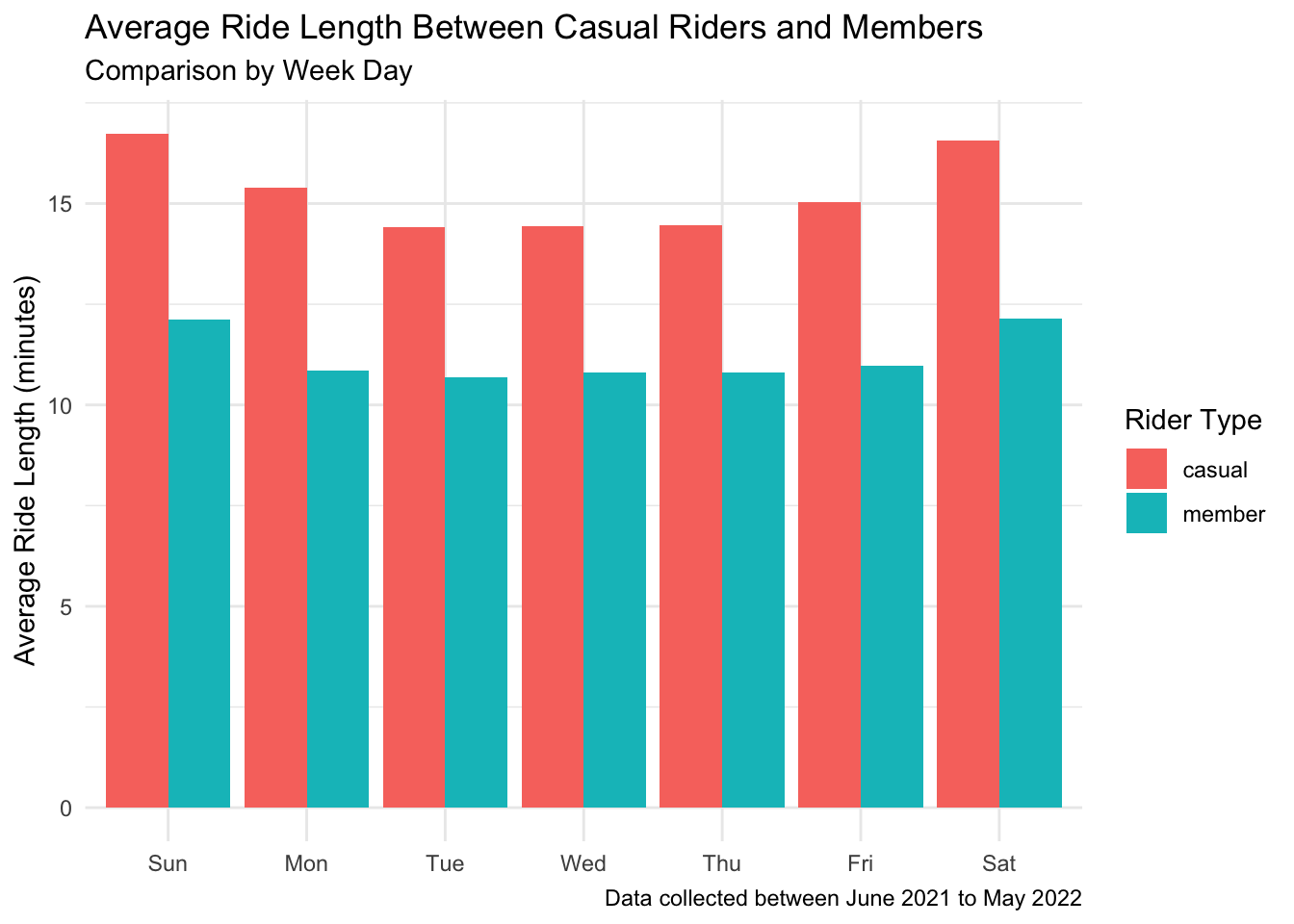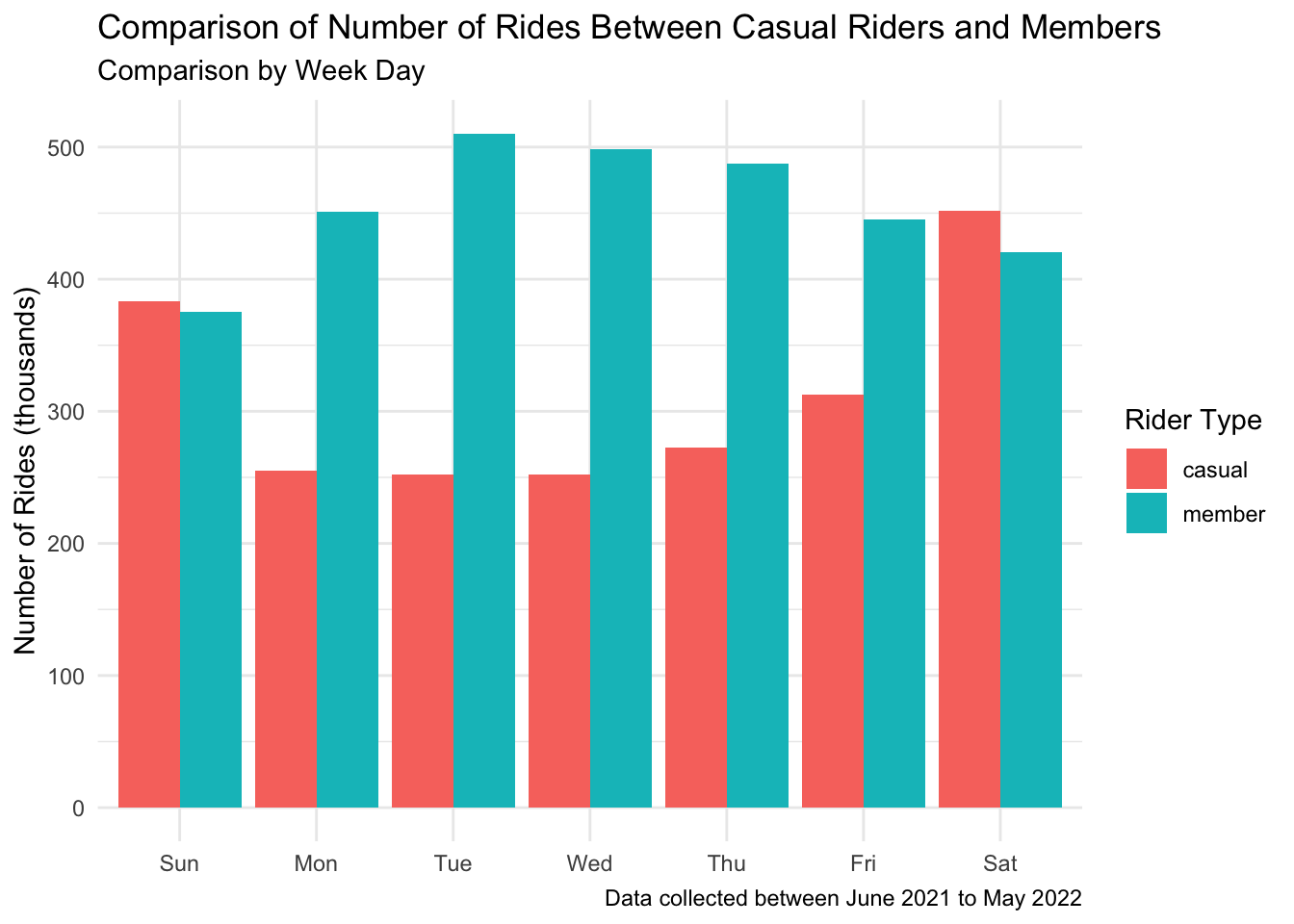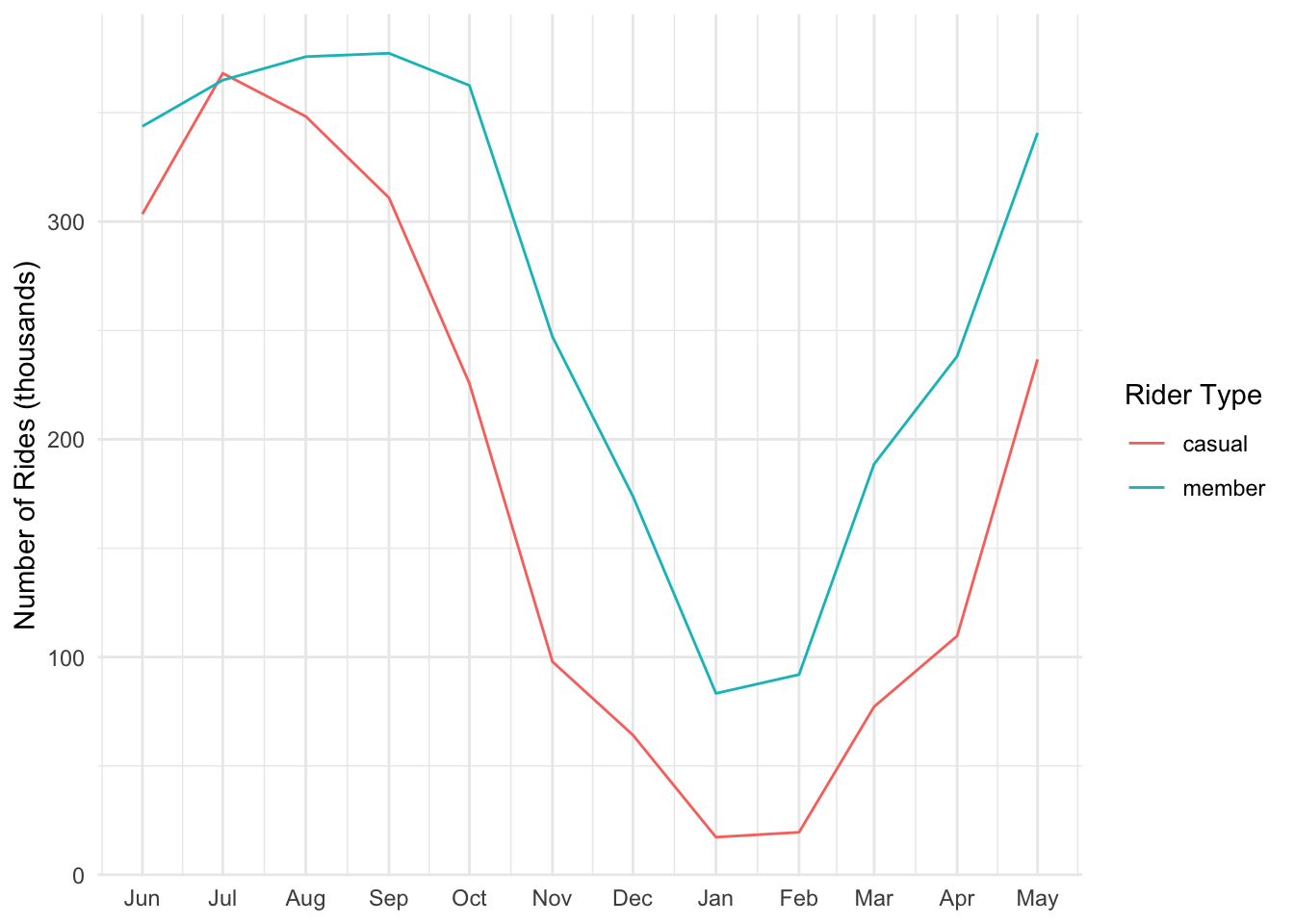# Case Study: CyclisticA Case Study analysing a fictional bike share company’s ride data that showcase all the skills required for Google’s Data Analytics Certification Capstone Project.

# The Project

## The Code

You are welcomed to browse how the data was cleaned, wrangled, analysed, and finally, visualized using R in my GitHub repository below:

Data Analysis - BikeShare

Continue scrolling to see me work through the analysis on R notebook.

Answering the Question: In what ways do members and casual riders use Divvy bikes differently?

## Setting up the environment

library(tidyverse)
library(janitor)
library(lubridate)
library(hms)
library(knitr)

The data is stored in csv files that are organized monthly.

# Loading the data
path <- "./divvy-tripdata/"
filenames <- list.files(path = path)

### Into a list

Since our data is being store in multiple csv files, I’ll be using a loop to store each data frame into a list. Doing so avoids cluttering up local environment.

all_df <- lapply(filenames, function(i) {
i <- paste(path,i,sep="")
})
filenames <- gsub("-","_",filenames)
names(all_df) <- gsub(".csv","",filenames)

### Wrangling the Data

joined_df <- all_df %>%
reduce(full_join)
## Joining, by = c("V1", "V2", "V3", "V4", "V5", "V6", "V7", "V8", "V9", "V10",
## "V11", "V12", "V13")
## Joining, by = c("V1", "V2", "V3", "V4", "V5", "V6", "V7", "V8", "V9", "V10",
## "V11", "V12", "V13")
## Joining, by = c("V1", "V2", "V3", "V4", "V5", "V6", "V7", "V8", "V9", "V10",
## "V11", "V12", "V13")
## Joining, by = c("V1", "V2", "V3", "V4", "V5", "V6", "V7", "V8", "V9", "V10",
## "V11", "V12", "V13")
## Joining, by = c("V1", "V2", "V3", "V4", "V5", "V6", "V7", "V8", "V9", "V10",
## "V11", "V12", "V13")
## Joining, by = c("V1", "V2", "V3", "V4", "V5", "V6", "V7", "V8", "V9", "V10",
## "V11", "V12", "V13")
## Joining, by = c("V1", "V2", "V3", "V4", "V5", "V6", "V7", "V8", "V9", "V10",
## "V11", "V12", "V13")
## Joining, by = c("V1", "V2", "V3", "V4", "V5", "V6", "V7", "V8", "V9", "V10",
## "V11", "V12", "V13")
## Joining, by = c("V1", "V2", "V3", "V4", "V5", "V6", "V7", "V8", "V9", "V10",
## "V11", "V12", "V13")
## Joining, by = c("V1", "V2", "V3", "V4", "V5", "V6", "V7", "V8", "V9", "V10",
## "V11", "V12", "V13")
## Joining, by = c("V1", "V2", "V3", "V4", "V5", "V6", "V7", "V8", "V9", "V10",
## "V11", "V12", "V13")
# Renaming the data frame
## reduce() function renamed the columns with some place holder values and placed the actual names into the first row of the data frame.
names(joined_df) <- joined_df[1,]
df <- joined_df %>%
slice(-1)

#### Let’s see what it’s like

str(df)
## 'data.frame':    5860776 obs. of  13 variables:
##  $ride_id : chr "99FEC93BA843FB20" "06048DCFC8520CAF" "9598066F68045DF2" "B03C0FE48C412214" ... ##$ rideable_type     : chr  "electric_bike" "electric_bike" "electric_bike" "electric_bike" ...
##  $started_at : chr "2021-06-13 14:31:28" "2021-06-04 11:18:02" "2021-06-04 09:49:35" "2021-06-03 19:56:05" ... ##$ ended_at          : chr  "2021-06-13 14:34:11" "2021-06-04 11:24:19" "2021-06-04 09:55:34" "2021-06-03 20:21:55" ...
##  $start_station_name: chr "" "" "" "" ... ##$ start_station_id  : chr  "" "" "" "" ...
##  $end_station_name : chr "" "" "" "" ... ##$ end_station_id    : chr  "" "" "" "" ...
##  $start_lat : chr "41.8" "41.79" "41.8" "41.78" ... ##$ start_lng         : chr  "-87.59" "-87.59" "-87.6" "-87.58" ...
##  $end_lat : chr "41.8" "41.8" "41.79" "41.8" ... ##$ end_lng           : chr  "-87.6" "-87.6" "-87.59" "-87.6" ...
##  $member_casual : chr "member" "member" "member" "member" ... ### Cleaning the Data Looks like all the data is imported as ‘character’ data type. Converting them to the correct types. ## Converting to correct data types, removing entries with NA df_clean <- df %>% mutate(started_at = ymd_hms(started_at)) %>% mutate(ended_at = ymd_hms(ended_at)) %>% mutate(start_lat = as.double(start_lat)) %>% mutate(start_lng = as.double(start_lng)) %>% mutate(end_lat = as.double(end_lat)) %>% mutate(end_lng = as.double(end_lng)) %>% mutate(ride_length = as_hms(difftime(ended_at, started_at)), .after = ended_at) %>% remove_empty(which = c("rows", "cols")) %>% na.omit() %>% filter(started_at<ended_at) %>% # remove cases where end time is somehow before or equals to the start time as_tibble() #### Calculating some basic stats ## Getting average amount of time different rider types ride df_stats <- df_clean %>% group_by(member_casual) %>% summarise(mean = as.duration(round(mean(ride_length))), max = as.duration(round(max(ride_length))), min = as.duration(round(min(ride_length))), sd = as.duration(round(sd(ride_length))), median = as.duration(round(median(ride_length))) ) kable(df_stats) member_casual mean max min sd median casual 1672s (~27.87 minutes) 3356649s (~5.55 weeks) 1s 12789s (~3.55 hours) 915s (~15.25 minutes) member 767s (~12.78 minutes) 89996s (~1.04 days) 1s 1187s (~19.78 minutes) 547s (~9.12 minutes) Wow.. the longest ride is 5 weeks?! There’s definitely some outliers here. Probably someone who took out a bike and forgot to return it (o_o”) #### Applying some statistics to help filter out the outliers df_quantile <- df_clean %>% group_by(member_casual) %>% summarise(q2 = as.duration(quantile(ride_length, 0.25)), q3 = as.duration(quantile(ride_length, 0.5)), q4 = as.duration(quantile(ride_length, 0.75)), iqr = as.duration(IQR(ride_length)), lower = q2-1.5*iqr, min = as.duration(round(min(ride_length))), upper = q4+1.5*iqr, max = as.duration(round(max(ride_length))) ) kable(df_quantile) member_casual q2 q3 q4 iqr lower min upper max casual 520s (~8.67 minutes) 915s (~15.25 minutes) 1668s (~27.8 minutes) 1148s (~19.13 minutes) -1202s (~-20.03 minutes) 1s 3390s (~56.5 minutes) 3356649s (~5.55 weeks) member 317s (~5.28 minutes) 547s (~9.12 minutes) 948s (~15.8 minutes) 631s (~10.52 minutes) -629.5s (~-10.49 minutes) 1s 1894.5s (~31.58 minutes) 89996s (~1.04 days) ### Noting down some ideas • Usage trends by month • Usage trends by time of day • Difference in ride distance df_filter <- df_clean %>% subset(select = c(ride_id, rideable_type, started_at, ended_at, ride_length, member_casual)) %>% filter(as.duration(ride_length)<df_quantile$upper) %>%
mutate(date = as.Date(started_at)) %>%
mutate(year_month = ym(format(as.Date(started_at), "%Y-%m"))) %>%
mutate(month = month(date)) %>%
mutate(day = day(date)) %>%
mutate(year = year(date)) %>%
mutate(day_of_week = wday(date)) %>%
mutate(weekday = wday(day_of_week, label = TRUE))
## Getting average amount of time different rider types ride after filtering outliers
df_stats2 <- df_filter %>%
group_by(member_casual) %>%
summarise(mean = as.duration(round(mean(ride_length))),
max = as.duration(round(max(ride_length))),
min = as.duration(round(min(ride_length))),
sd = as.duration(round(sd(ride_length))),
median = as.duration(round(median(ride_length)))
)
kable(df_stats2)

member_casual mean max min sd median
casual 929s (~15.48 minutes) 3389s (~56.48 minutes) 1s 624s (~10.4 minutes) 781s (~13.02 minutes)
member 669s (~11.15 minutes) 3389s (~56.48 minutes) 1s 500s (~8.33 minutes) 528s (~8.8 minutes)

# Descriptive Analysis

## Ridership by type and weekday

df_filter %>%
aggregate(ride_length ~ member_casual + weekday, FUN = mean) %>%
kable(col.names = c("Type", "Weekday", "Ride Length"))
Type Weekday Ride Length
casual Sun 1004.4653 secs
member Sun 727.8924 secs
casual Mon 923.1539 secs
member Mon 650.9789 secs
casual Tue 864.3906 secs
member Tue 641.2614 secs
casual Wed 865.7356 secs
member Wed 647.6209 secs
casual Thu 868.0591 secs
member Thu 648.5437 secs
casual Fri 902.5364 secs
member Fri 657.8947 secs
casual Sat 994.1535 secs
member Sat 728.4653 secs

## Visualizing it

df_ride_weekday <- df_filter %>%
group_by(member_casual, weekday) %>%
summarise(rides = n(), average = mean(ride_length)) %>%
arrange(member_casual, weekday)
## summarise() has grouped output by 'member_casual'. You can override using the
## .groups argument.
df_ride_weekday %>%
ggplot(aes(x = weekday, y = average/60, fill = member_casual)) +
geom_col(position = "dodge") +
theme_minimal() +
labs(title = "Average Ride Length Between Casual Riders and Members",
subtitle = "Comparison by Week Day",
y = "Average Ride Length (minutes)",
x = NULL,
fill = "Rider Type",
caption = "Data collected between June 2021 to May 2022")
## Don't know how to automatically pick scale for object of type difftime. Defaulting to continuous.Casual riders consistently seem to rent the bikes for longer than members.

df_ride_weekday %>%
ggplot(aes(x = weekday, y = rides/1000, fill = member_casual)) +
geom_col(position = "dodge") +
theme_minimal() +
labs(title = "Comparison of Number of Rides Between Casual Riders and Members",
subtitle = "Comparison by Week Day",
y = "Number of Rides (thousands)",
x = NULL,
fill = "Rider Type",
caption = "Data collected between June 2021 to May 2022")Members ride a lot more on weekdays compared to Casual riders who edge out more rides on weekends.

# Visualizing the number of rides per month

df_filter %>%
group_by(member_casual, year_month) %>%
tally() %>%
ggplot() +
geom_line(aes(x = year_month, y = n/1000, color = member_casual)) +
theme_minimal() +
scale_x_date(date_breaks = "1 month", date_labels = "%b") +
labs(x = NULL, y = "Number of Rides (thousands)", color = "Rider Type")Both types of riders seem to follow the same monthly riding trends. Most riders are not fond of riding during winter months.

df_filter %>%
group_by(member_casual, year_month) %>%
summarise(average_ride = mean(as.numeric(as.duration(ride_length), "minutes"))) %>%
ggplot() +
geom_line(aes(x = year_month, y = average_ride, color = member_casual)) +
theme_minimal() +
scale_x_date(date_breaks = "1 month", date_labels = "%b") +
scale_y_continuous(limits = c(0, 30)) +
labs(title = "Monthly Average Ride Length per Month", x = NULL, y = "Average Ride Length (minutes)", color = "Rider Type")
## summarise() has grouped output by 'member_casual'. You can override using the
## .groups argument.